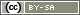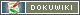# calcMean(), calcSD(), ...

These functions perform simple statistical calculations within the questionnaire, they all follow the same syntax:

`calcXYZ(array Data, [boolean Filter])`

• Data
An array of numeric values. The values `false` and `null` are ignored in the calculation. Numbers that are stored as strings are converted to numbers.
• Filter
If this option is set to `true`, negative values are ignored in the calculation. This is useful if raw data from SoSci Survey are used. Missing values (no response and alternative options) are coded with negative codes.

The function returns either a floating point value (float) or `NULL` if the calculation fails (e.g. because there are no valid values).

The following functions are available:

• `calcMean()` Calculate arithmetic mean value
• `calcSD()` Calculate standard deviation (1/n)
• `calcESD()` Calculate empirical standard deviation (1/(n-1), see. sample variance)

Tip: If you want to directly calculate with the answers of the participant, use the functions `valueMean()`, `valueSD()`, `valueVar()`.